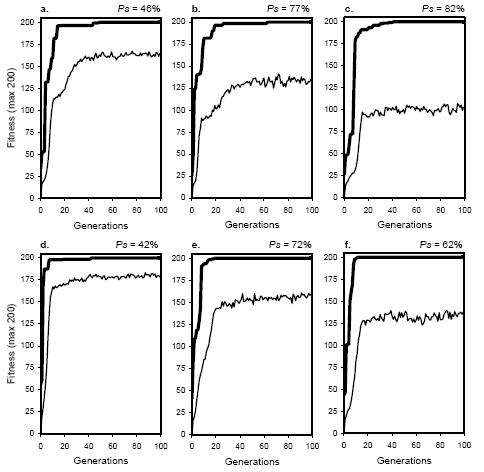Home
News
Author
Q&A
Tutorials
GEP Biblio
Contacts

Visit Gepsoft

 The evolutionary dynamics of transposition are shown in Figure 7.3. As you can see, the dynamics obtained for both RIS and IS transposition are similar to the ones obtained for mutation. And this is worth pointing out, because not all operators display this kind of dynamics (see the dynamics of recombination in the next section). Like in mutation, the gap between average fitness and best fitness increases with transposition rate. Also worth pointing out is that populations are evolving more efficiently at pris = 1.0 (Ps = 82%) than at pis = 1.0 (Ps = 62%). Consequently, the gap between average and best fitness is smaller in the last case (compare plots c and f). More generally, the plots for average fitness obtained for RIS transposition occupy lower positions than the corresponding IS dynamics. As previously shown, for healthy populations, this is an indicator of a more efficient evolution. Indeed, as shown in Figure 7.1, RIS transposition performs slightly better than IS transposition.Figure 7.3. Evolutionary dynamics characteristic of populations evolving by RIS transposition (plots a, b, and c) and IS transposition (plots d, e, and f). The success rate above each plot was determined in the experiment of Figure 7.1. a) pris = 0.2. b) pris = 0.6. c) pris = 1.0. d) pis = 0.2. e) pis = 0.6. f) pis = 1.0. Note that the evolutionary behavior is similar to mutation (see Figure 7.2) and very different from recombination (see Figure 7.4). Note also that the plots for average fitness obtained for RIS transposition occupy lower positions than the corresponding IS dynamics, an indicator of a more tumultuous and, in this case, more efficient evolution. The fact that RIS and IS transposition exhibit a behavior similar to mutation further reinforces the uniqueness of recombination. We will see in the next section that populations undergoing recombination alone behave very strangely: they evolve very smoothly without appreciable oscillations on average fitness and are constantly trying to close the gap between average and best fitness.